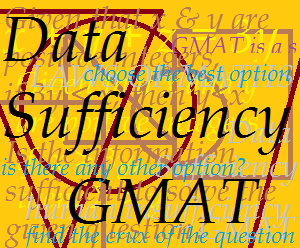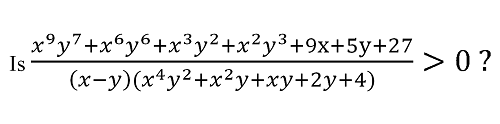## Tackle Options in GMAT DS Questions the Oak’s Academy Way

~ by our Quantitative Reasoning Faculty

Step 2 of the Approach to DS Questions: Tackle the Options the Oak’s Way

Step 1 of the approach dealt with carefully reading the question statement (see previous blog). Once that is done you have to deal with the options, which are standard in DS questions:(A) Statement (1) alone is sufficient but statement (2) alone is not sufficient to answer the question asked

(B) Statement (2) alone is sufficient but statement (1) alone is not sufficient to answer the question asked

(C) Both statements (1) and (2) together are sufficient to answer the question asked, but neither statement alone is sufficient

(E) Statements (1) and (2) together are not sufficient to answer the question asked and additional data are needed.

To deal with them in the most systematic way possible just follow the sufficiency/insufficiency table below. It offers the best way of thinking through the options

What’s so great about the Oak’s Way?

What it makes the sufficiency/insufficiency table so cool is that once you’ve found the crux of the question, it will help you to zero in on the answer quickly and efficiently. The essence of the approach is to go step-by-step i.e. first, check STATEMENT (1) for sufficiency/insufficiency and then, do the same for STATEMENT (2). The table below gives you the answer you should choose (last column) depending on whether Statements (!) and (2) are sufficient or insufficient (first and second columns)

So, how does it work in an actual question? Let’s have a look at the previous question again.

If x and y are distinct positive integers then:(1) x = 2 (y + 3)

(2) x2 = y2 + 4

Working with Statement (1)

Now, we already know that the only information required to reach the final answer is whether x – y > 0.

According to the GMAT approach we first consider statement (1) that is, x = 2(y + 3) alone.

If we simplify it, we get:

x = 2y + 6

x – 2y = 6.

This tells us that x – y has to be positive (given that x and y are positive integers, in x – y, we are subtracting a smaller value from x than in x – 2y).

Thus, statement (1) alone leads us to conclusion that x – y is must be positive, that is, x – y > 0 and hence, it is sufficient to reach the final answer. This means that our answer has to be either (A) Statement (1) alone is sufficient but statement (2) alone is not sufficient to answer the question asked’ or, (D) ‘Each statement alone is sufficient to answer the question asked’.

Working with Statement (2)

To choose between them, we consider statement (2) i.e. x2 = y2 + 4 alone.

If we simplify this, we get:

x2 – y2 = 4

(x + y) (x – y) = 4

Of the two factors we’ve on the left hand side of this equation, (x + y) is always going to be positive as it is an addition of two positive integers and if the product (x + y) (x – y) is 4 (i.e. positive) we can easily conclude that

(x – y) has to be positive! And that again serves our purposes! If x – y is positive, the entire expression in the question definitely has to be positive.

Thus, statement (2) alone is also sufficient and hence, the answer is option (D)!

In this way by using the GMAT approach to the options and making some simple observations, an apparently super complex DS question becomes easy to solve.

Practice Question

Now, try this question.

Given that a, b, c, d, e are positive integers and that ‘b’ is an odd integer, is the product (a+b)(a+c)(a+d)(a+e) an odd integer?

(1) a is an odd integer

(2) c is an even integer